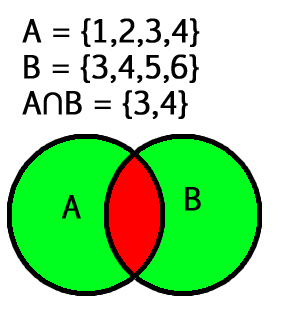# Intersection() function Python

## Intersection() function Python

Python intersection() function return a new set with an element that is common to all set

The intersection of two given sets is the largest set which contains all the elements that are common to both sets. The intersection of two given sets A and B is a set which consists of all the elements which are common to both A and B.### Examples of intersection:

Input: Let set A = {2, 4, 5, 6}

and set B = {4, 6, 7, 8}

Output: {4,6}

Explanation: Taking the common elements in both the sets, we get {4,6} as the intersection of both the sets.

### Python intersection() Syntax:

set1.intersection(set2, set3, set4….)
In parameters, any number of sets can be given

### Python intersection()Return value:

The intersection() function returns a set, which has the intersection of all sets(set1, set2, set3…) with set1. It returns a copy of set1 only if no parameter is passed.

## Python intersection() Example

### Example 1: Working of set intersection()

 `# Python3 program for intersection() function` `set1 ``=` `{``2``, ``4``, ``5``, ``6``}` `set2 ``=` `{``4``, ``6``, ``7``, ``8``}` `set3 ``=` `{``4``, ``6``, ``8``}` `# union of two sets` `print``(``"set1 intersection set2 : "``,` `      ``set1.intersection(set2))` `# union of three sets` `print``(``"set1 intersection set2 intersection set3 :"``,` `      ``set1.intersection(set2, set3))`

Output:

```set1 intersection set2 :  {4, 6}
set1 intersection set2 intersection set3 : {4, 6}```

### Example 2: Python set intersection operator(&)

we can also get intersection using & operator.

 `# Python3 program for intersection() function` `set1 ``=` `{``2``, ``4``, ``5``, ``6``}` `set2 ``=` `{``4``, ``6``, ``7``, ``8``}` `set3 ``=` `{``1``, ``0``, ``12``}` `print``(set1 & set2)` `print``(set1 & set3)` `print``(set1 & set2 & set3)`

Output:

```{4, 6}
set()
set()```

### Example 3: Python set intersection opposite

symmetric_difference() is a opposite to the set.intersection() methods.

 `# Python3 program for intersection() function` `set1 ``=` `{``2``, ``4``, ``5``, ``6``}` `set2 ``=` `{``4``, ``6``, ``7``, ``8``}` `set3 ``=` `{``1``, ``0``, ``12``}` `print``(set1.symmetric_difference(set2))` `print``(set1.symmetric_difference(set3))` `print``(set2.symmetric_difference(set3))`

Output:

```{2, 5, 7, 8}
{0, 1, 2, 4, 5, 6, 12}
{0, 1, 4, 6, 7, 8, 12}```

### Example 4: Python set intersection empty

 `set1 ``=` `{}` `set2 ``=` `{}` `# union of two sets` `print``(``"set1 intersection set2 : "``,` `      ``set``(set1).intersection(``set``(set2)))`

Output:

`set1 intersection set2 :  set()`

Last Updated on March 1, 2022 by admin

## Function Annotations in PythonFunction Annotations in Python

Function Annotations in Python PEP: PEP stands for Python Enhancement Proposal. It is a design document

## First Class functions in PythonFirst Class functions in Python

First Class functions in Python First class objects in a language are handled uniformly throughout. They

## os.getpid() method – Pythonos.getpid() method – Python

Python | os.getpid() method OS module in Python provides functions for interacting with the operating system.

## How to Filter DataFrame Rows Based on the Date in Pandas?How to Filter DataFrame Rows Based on the Date in Pandas?

How to Filter DataFrame Rows Based on the Date in Pandas? Different regions follow different

## Python – Draw Star Using Turtle GraphicsPython – Draw Star Using Turtle Graphics

Python – Draw Star Using Turtle Graphics In this article, we will learn how to

## Generating all possible Subsequences using RecursionGenerating all possible Subsequences using Recursion

Generating all possible Subsequences using Recursion Given an array. The task is to generate and

## Play a video using OpenCVPlay a video using OpenCV

Python | Play a video using OpenCV OpenCV (Open Source Computer Vision) is a computer vision